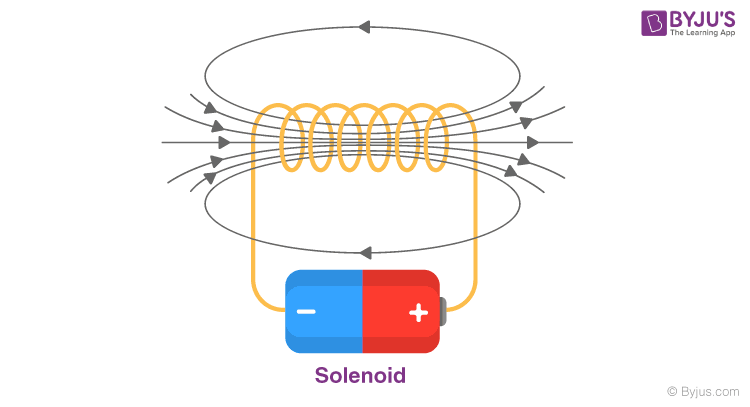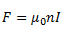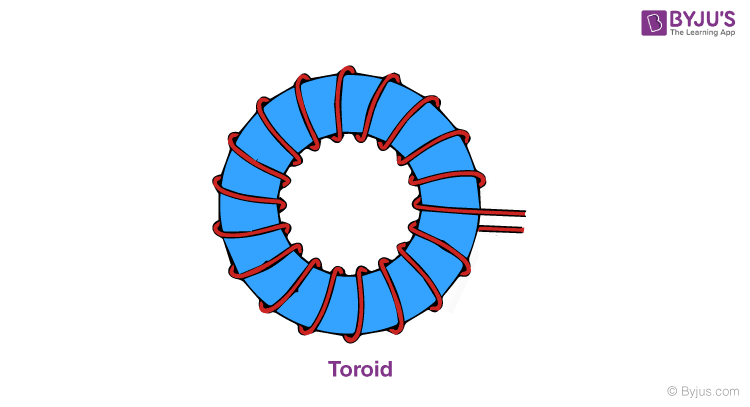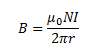Jet Set Go! All about Aeroplanes Jet Set Go! All about Aeroplanes

# Solenoid and Toroid

## What Is Solenoid?

Let us consider a solenoid, such that its length is large as compared to its radius. Here, the wire is wound in the form of a helix with a very little gap between any two turns. Also, the wires are enameled, thus rendering them insulated from each other. As a result, each turn can be taken as a closed circular loop. The magnetic field thus generated is equivalent to that generated by a circular loop and the total magnetic field generated by the solenoid can be given as the vector sum of force generated by each such turn. The magnetic field lines generated inside a finite solenoid has been shown in the figure below.We can see from the figure that the magnetic field inside the solenoid is uniform in nature and is along the axis of the solenoid. The field at the exterior at any point immediately to the solenoid is very weak and the field lines cannot be seen near the close vicinity. It is important to note that the field inside it is parallel to its axis at every position.

From the Ampere’s Law, the magnetic force produced by a solenoid can be given as,Where n is the number of turns of the wire per unit length, I is the current flowing through the wire and the direction is given using the right-hand thumb rule.

## To Know More about Solenoids, Click on the Video Below## What Is Toroid?

A toroid is shaped like a solenoid bent into a circular shape such as to close itself into a loop-like structure. The toroid is a hollow circular ring, as can be seen in the image shown below, with many turns of enameled wire, closely wound with negligible spacing between any two turns.The magnetic field inside and outside the toroid is zero. The magnetic field inside the toroid, along with the circular turn, is constant in magnitude and its direction inside the toroid is clockwise as per the right-hand thumb rule for circular loops.

The magnetic field due to a toroid can be given as,Where N is the number of turns of the toroid coil, I is the amount of current flowing and r is the radius of the toroid.

## Accelerate Your JEE Main & Advanced Exam Preparation by Watching this Video about Magnetism and Force on Dielectric in Charged Capacitor## Frequently Asked Questions – FAQs

### What is a solenoid?

A solenoid is a kind of electromagnet created using a helical wire whose length is much larger than its diameter, which produces controlled magnetic fields. The coil can generate uniform magnetic fields in space when an electric current is flowing through it.

### What is a toroid?

A toroid is similar to a solenoid bent into a disc shape in order to close itself into a loop-like form. It is a hollow disc-shaped ring with numerous turns of wire (enamelled), tightly wound with minute spacing between any two turns.

### What is a magnetic field?

A magnetic field is a field that shows the magnetic influence on magnetic materials, moving electric charges, and electric currents.

### What is the value of the magnetic field inside a toroid?

The magnetic field inside and outside the toroid is zero.

### Who invented the toroid?

André-Marie Ampère invented the solenoid.

## Watch the Video and Learn about Magnetic Field Due to Solenoid and Properties of Magnetic Field Lines